## Partitions, perforations and tilings

We are “geometric” in our thinking – perhaps, we are hence a little more Greek or the old type Arya than the later Hindu (who is more algebraic) in mentality. Long back in college we were fascinated by implicit trigonometric relations but were utterly defeated by the difficulty in visualizing. Without visualization, of what use are these relations to one who is inclined towards visible geometry? Then we wrote a bit of code that allowed us to visualize the same. Today far more efficient programs exist to do the same and we tend to revisit these relations, perhaps as a reenactment of our childish fancies.

During those explorations of ours we realized that certain class of trigonometric relations exist which produce the following:
1) Partitions: These might be defined as divisions of the x-y plane into continuous zones of 2 colors.
2) Perforations: These can be defined as completely bounded zones which can be visualized as perforations of the x-y plane.
Now, there might be relations which generate both partitions and perforations at the same time.
3) Depressions and bosses: They might be visualized as incomplete precursors of perforations in the x-y plane with the plane being deformed in either direction by vectors perpendicular to it.
4) Tilings: These completely tile the x-y plane by means of bounded tiles that share edges. There might be tiles which bear perforations or depressions and bosses on them.
5) Loopings: These might be defined as adjacent perforations that become contiguous via intersections involving a single point.

To illustrate these let us consider the below relationship:$cot(cos^{3} (x-y)+sin^{2} (x+y))>|sin(x+y)-cos(x-y)|$
It produces the below figure below which includes both partitions and perforations (the rhombuses with concave sides).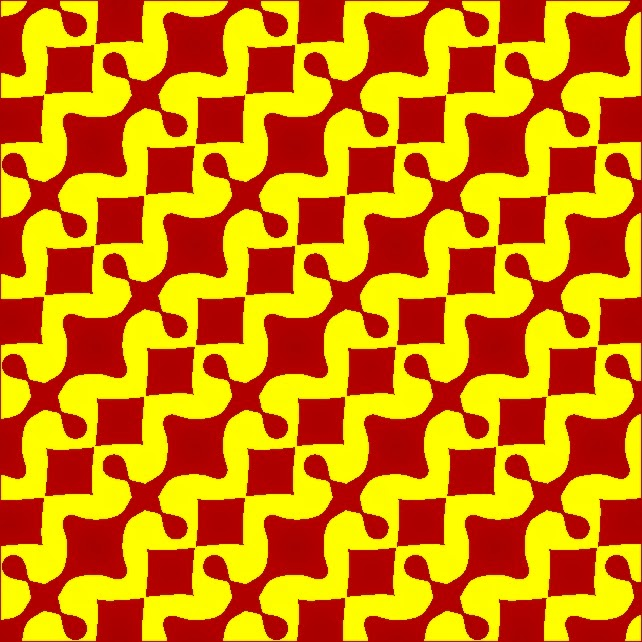Some of these can have highly twisted partitions, in this case combined with depressions and bosses, that superficially make it look like a tiling (the figure to the right) as produced by:$tan(13cos^{3}(x-y)+17sin^{3}(x+y))>|sin(x+y)-cos(x-y)|$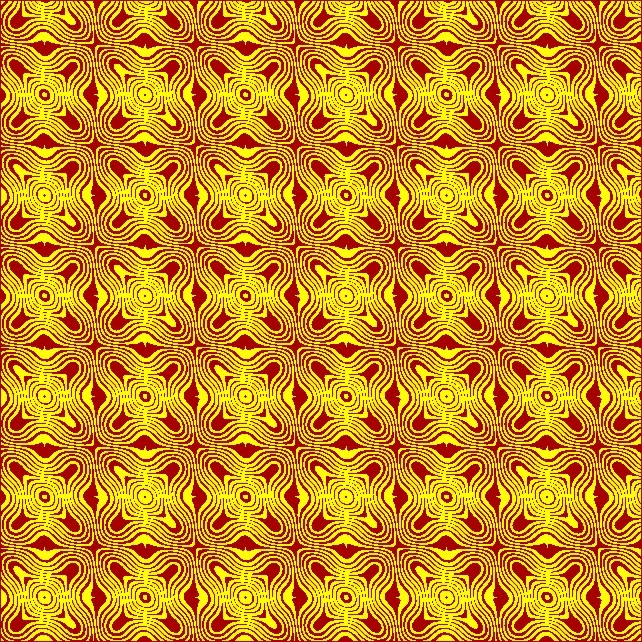Now let us consider the relation:$sin(9sin^{2}(x+y) \pm 12cos^{2}(x-y))>|sin(x+y)-cos(x-y)|$
It produces perforations that show some complexity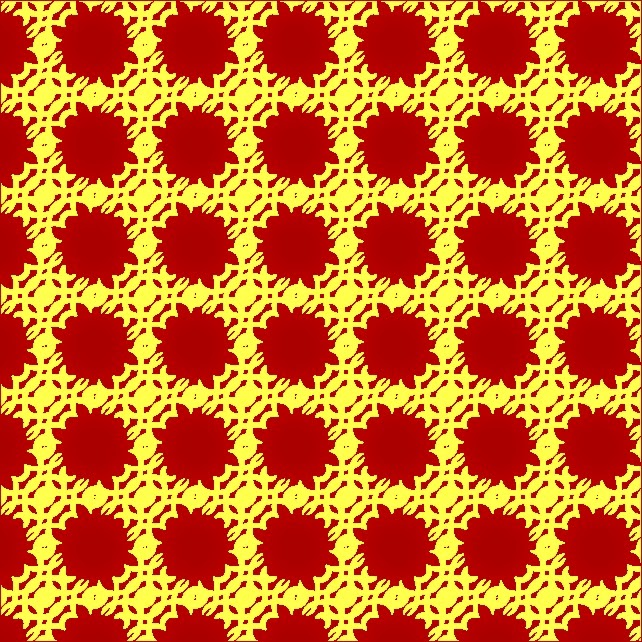Now let us consider the relation:$cot(cos(x-y)+sin(x+y))>|sin(x+y)-cos(x-y)|$
This produces a tiling but the tiles respectively have depressions or bosses.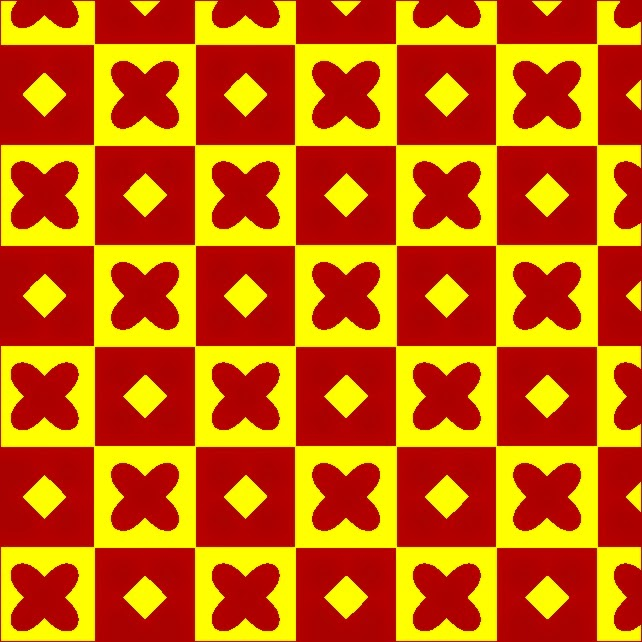The introduction of an irrational constant like$\phi$ adds a new wrinkle to these relations. For example let us consider:$sec(9sin^{8}(x+\phi y)+4cos^{8}(\phi x-y))>|sin(x+y)-cos(x-y)|$
The Golden ratio$\phi=1.61803398875$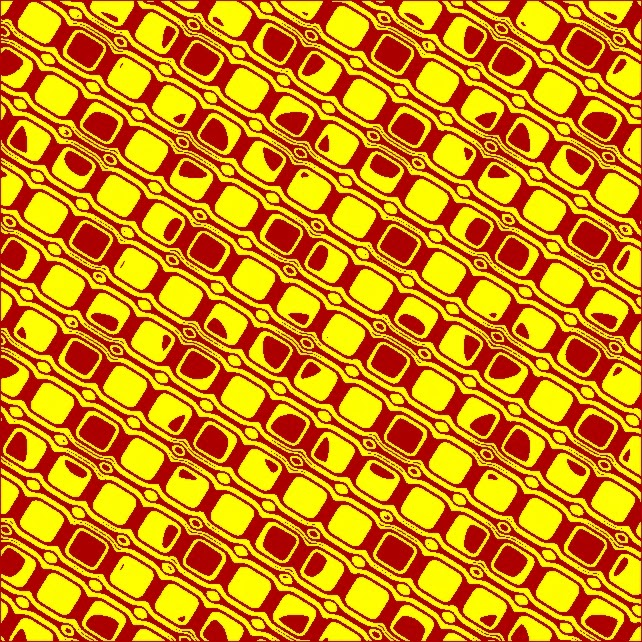The irrationality of the constant manifests in the resultant partitions, perforations, depressions/bosses in form an endless diversity of patterns – i.e. you do not get the same thing again even though they all look similar. This discovery greatly pleased us when we first stumbled upon it.

We shall conclude with a foray into the realm of personal symbolism. Below is what we term the gaze of indra intertwined with the gaze of Odin; produced by:$cot(4sin^{2}(x+y)+13cos^{2}(x-y))>|sin(x+y)-cos(x-y)|$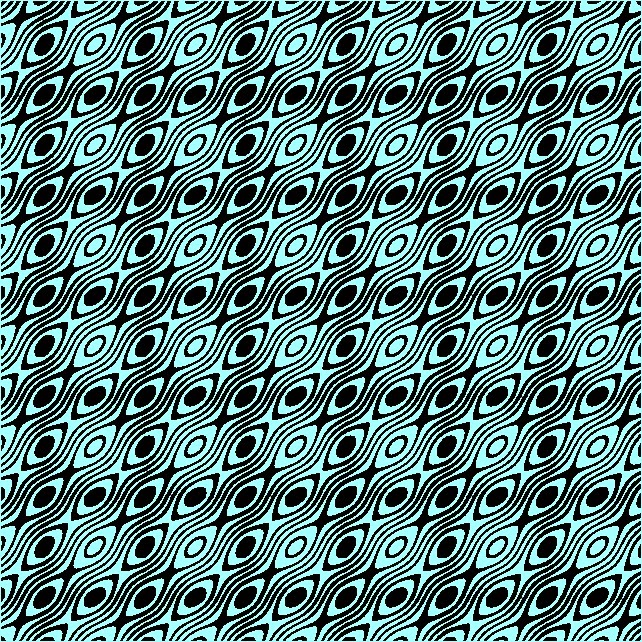Why do we call it so? In Hindu tradition indra is said to have a 1000 eyes with which he watches over all. Hence, those many rows of eyes gazing at us are those of indra. Now in Germanic lore Odin is said to have sacrificed an eye of his at the well of Mimir. While he was left with just one eye, the drink at the well made him all knowing. Hence, we depict the second set of rows as representing the all-knowing gaze of Odin with only one eye being function and the other blind. The ocular anomaly is something he shares with his Hindu cognate rudra. While rudra is typically known as three-eyed, he is also described as being one-eyed in the rudra rahasya-gAna of the sAmaveda. This ocular trait is inherited by a subset of the ectypes of rudra known as the vidyeshvara-s who form part of the saiddhAntika shaiva pantheon. As an aside one may also note the “black grid” optical illusion produced by it.

The next one is termed the gaze of bhaga and aryaman and is generated by:$cot(3sin^{2}(x+y)+cos^{3}(x-y))>|sin(x+y)-cos(x-y)|$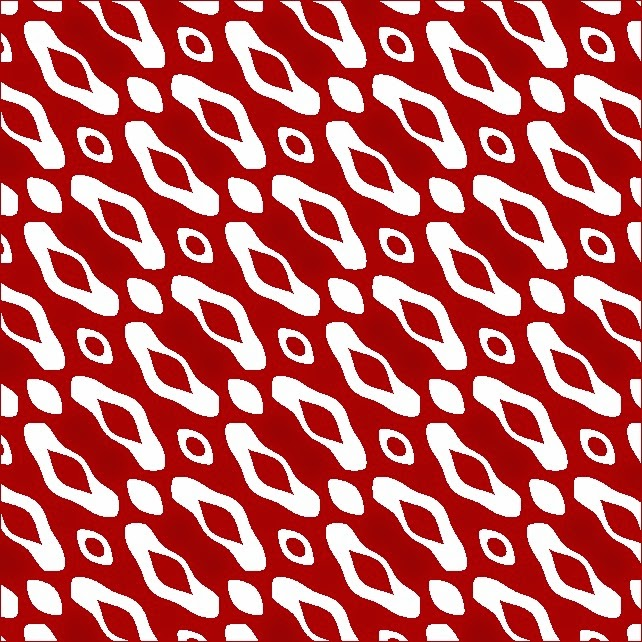Here there are two sets of quasi-symmetric face-like figures with eyes and a mouth. Of these aryaman is the far-seeing one with normal eyes, while bhaga is the one with blind eyes. Hindu tradition going back to the shruti records a legend of bhaga losing his eyes when rudra attacked the yaj~na or prajApati. In the mahAbhArata the heroes are cast in the image of the ancient gods. In this matrix some of them are very obvious in their resemblance to the gods. Among the non-obvious ones are vidura who is cast in the image of the all-seeing aryaman, who is also the regent of the homestead, and the blind dhR^itarAShTra who is cast in the image of bhaga. Among the Aditya-s the first and the most prominent pair are mitra and varuNa, they are followed by the pair aryaman and bhaga. The former pair is potentially partitioned between pANDu and yudhiShThira in the mahAbhArata.

This entry was posted in art, Life and tagged , , , . Bookmark the permalink.